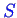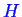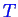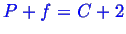Next: Phase Diagram Construction Up: Continuum Thermodynamics Previous: Le Chatelier's Principle

## Gibbs-Duhem Equation and its Consequences

You might have noticed that we have defined the Gibbs free energy in two different ways above, once by subtracting off combinations of,, andand once as a sum of chemical potentials and amounts of species. The fact that they are equal gives a new useful relation called the ``Gibbs-Duhem Relation.'' The Gibbs-Duhem relation allows us to calculate relationships between quantities as a system remains in equilibrium. One example is the Clausius-Clapeyron equation that relates equilibrium changes in pressure to changes in temperature as a function of material parameters. These relationships restrict the degrees of freedom in a system at equilibrium. These restrictions make up the famous ``Gibbs Phase Rule''. The Gibbs phase rule tells you how many things you can change and still have equilibrium and it depends on the number of chemical components and the number of existing phases. You will all know Gibbs phase rule by the end of the class--I hope I can also teach you what it means.Next: Phase Diagram Construction Up: Continuum Thermodynamics Previous: Le Chatelier's Principle
W. Craig Carter 2002-09-05